# RD Sharma Solutions - Chapter 2 - Powers (Ex-2.1) - Class 8 Math Notes | Study RD Sharma Solutions for Class 8 Mathematics - Class 8

## Class 8: RD Sharma Solutions - Chapter 2 - Powers (Ex-2.1) - Class 8 Math Notes | Study RD Sharma Solutions for Class 8 Mathematics - Class 8

The document RD Sharma Solutions - Chapter 2 - Powers (Ex-2.1) - Class 8 Math Notes | Study RD Sharma Solutions for Class 8 Mathematics - Class 8 is a part of the Class 8 Course RD Sharma Solutions for Class 8 Mathematics.
All you need of Class 8 at this link: Class 8

#### Question 1: Express each of the following as a rational number of the form p/q,pq, where p and q are integers and q ≠ 0.

(i) 2−3
(ii) (−4)−2

(iii)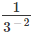(iv)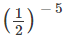12-5
(v)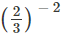#### Answer 1: We know that a−n =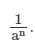Therefore,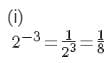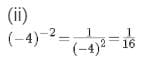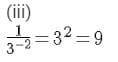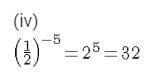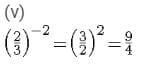#### Question 2: Fiind the value of each of the following:(i) 3−1 + 4−1(ii) (30 + 4−1) × 22(iii) (3−1 + 4−1 + 5−1)0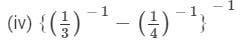(i) We know from the property of powers that for every natural number a, a−1 = 1/a. Then: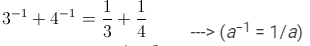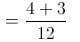= 7/12

(ii) We know from the property of powers that for every natural number aa−1 = 1/a.
Moreover, a0 is 1 for every natural number a not equal to 0. Then:

(30+41)×22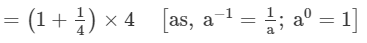=5/4 ×4

= 5

(iii) We know from the property of powers that for every natural number aa−1 = 1/a.
Moreover, a0 is 1 for every natural number a not equal to 0. Then:
(31+41+51)=1(3-1+4-1+5-1)=1          ---> (Ignore the expression inside the bracket and use a0 = 1 immediately.)

(iv) We know from the property of powers that for every natural number aa−1 = 1/a. Then: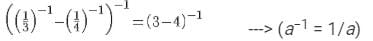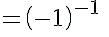=1-1                       ---> (a−1 = 1/a)

#### Question 3:Find the value of each of the following: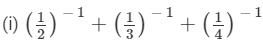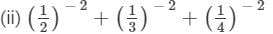(iii) (2−1 × 4−1) ÷ 2−2
(iv) (5−1 × 2−1) ÷ 6−1

(i)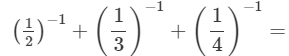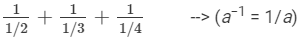(ii)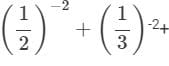14-2=11/22+11/32+11/42        --> (an = 1/(an))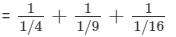--> ((a/b)n = (an/bn))

= 4+9+16
=29

(iii)
(2−1×4−1)÷2−2=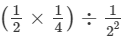--> (an = 1/(an))

=1/8×418×4

= 2

(iv)
(5−1×2−1)÷6−1=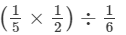--> (a−n = 1/(an))

= 1/10 × 6

= 3/5

#### Question 5: Simplify: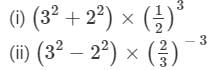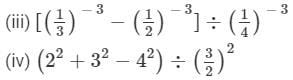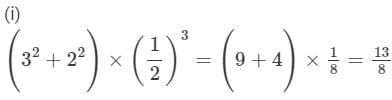(ii)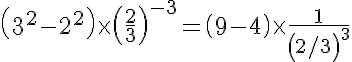---> (a−1=1/(an))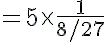---> ((a/b)n = (an)/(bn))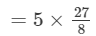135/8

(iii)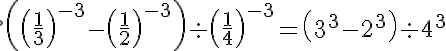--->(a-n = 1/(an))
(278)÷64(27-8)÷64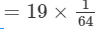= 19/64

(iv)
(22+3242)÷(32)2=(4+916)× 9/4   ---> ((a/b)n = (an)/(bn))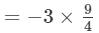= -27/4

#### Answer 7: Using the property a−1 = 1/a for every natural number a, we have (1/2)−1 = 2 and (−4/7)−1 = −7/4. We have to find a number x such that

2x= -7/4

Dividing both sides by 2, we get:
x=-7/8

Hence, the required number is −7/8.

####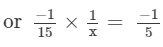or x = 1/3

Hence, (−15)−1 should be divided by 1/313 to obtain (−5)−1.

#### Question 9: Write each of the following in exponential form:(i) (3/2)−1×(3/2)−1×(3/2)−1×(3/2)−132-1×32-1×32-1×32-1(ii) (2/5)−2×(2/5)−2×(2/5)−225-2×25-2×25-2

(i) (3/2)−1×(3/2)−1×(3/2)−1×(3/2)−1=(3/2)−1+(−1)+(−1)+(−1)                {am×an=am+n
=(3/2)−4
(ii) (2/5)−2×(2/5)−2×(2/5)−2=(2/5)−2+(−2)+(−2)                {am×an=am+n
=(2/5)−6

#### Question 10: Evaluate:(i) 5−2(ii) (−3)−2(iii) (1/3)−413-4(iv) (- 1/2)−1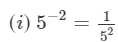---> (an = 1/(an))

= 1/25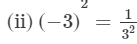---> (an = 1/(an))

= 1/9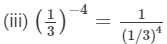---> (an = 1/(an))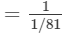= 81

(iv)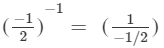---> (a−1 = 1/(a))

= -2

#### Question 11: Express each of the following as a rational number in the form p/q:pq:

(i) 6−1
(ii) (−7)−1

(iii) (1/4)114-1

(iv) (-4) -1 × (-3/2) -1

(v) (3/5) -1  ×( 5/2) -1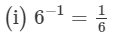---> (a−1 = 1/a)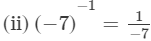---> (a−1 = 1/a)

-1/7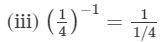---> (a−1 = 1/a)

= 4

(iv)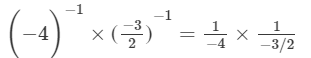---> (a−1 = 1/a)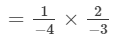= 1/6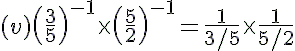---> (a−1 = 1/a)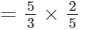= 2/3

#### Question 12: Simplify:

(i) {41×31}24-1×3-12
(ii) {51÷61}35-1÷6-13
(iii) (21+31)12-1+3-1-1
(iv) {31×41}1×513-1×4-1-1×5-1
(v) (4151)÷31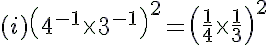---> (a−1 = 1/a)

= (1/12) 2

=(1)2/(12)2                --->((a/b)n = (an)/(bn))

= 1/144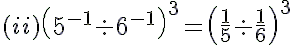---> (a−1 = 1/a)

= ( 6/5) 3

= 216/125  --->((a/b)n = (an)/(bn))

(iii) (21 + 31)1 =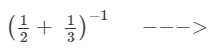−−−> (a1= 1/a)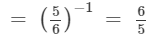−−−> (a1= 1/a)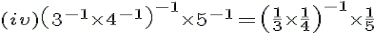---> (a−1 = 1/a)

=(1/12)1  × 1/5

=12×1/5   ---> (a−1 = 1/a)

12/5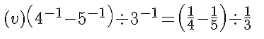---> (a−1 = 1/a)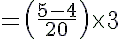=1/20 ×3=120×3

= 3/20

(i) (1/4)3143

(ii) 3535